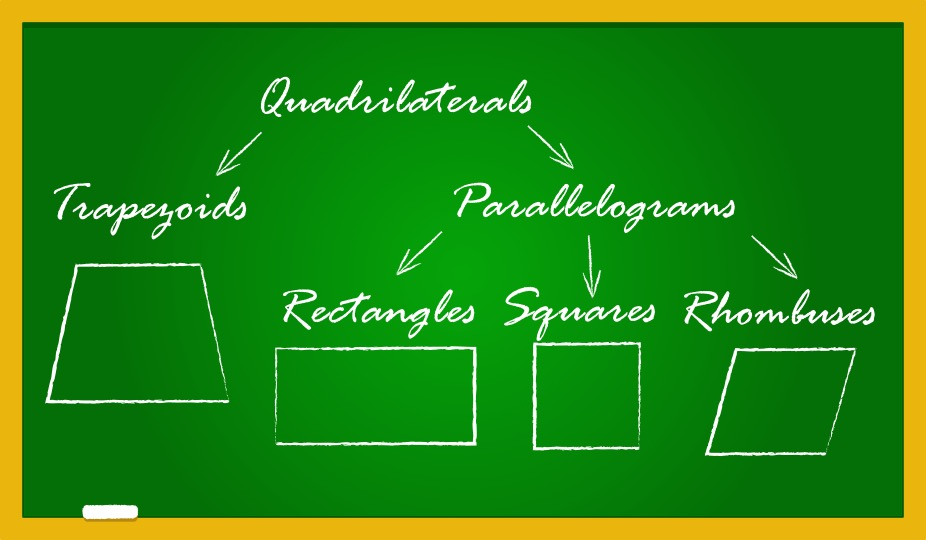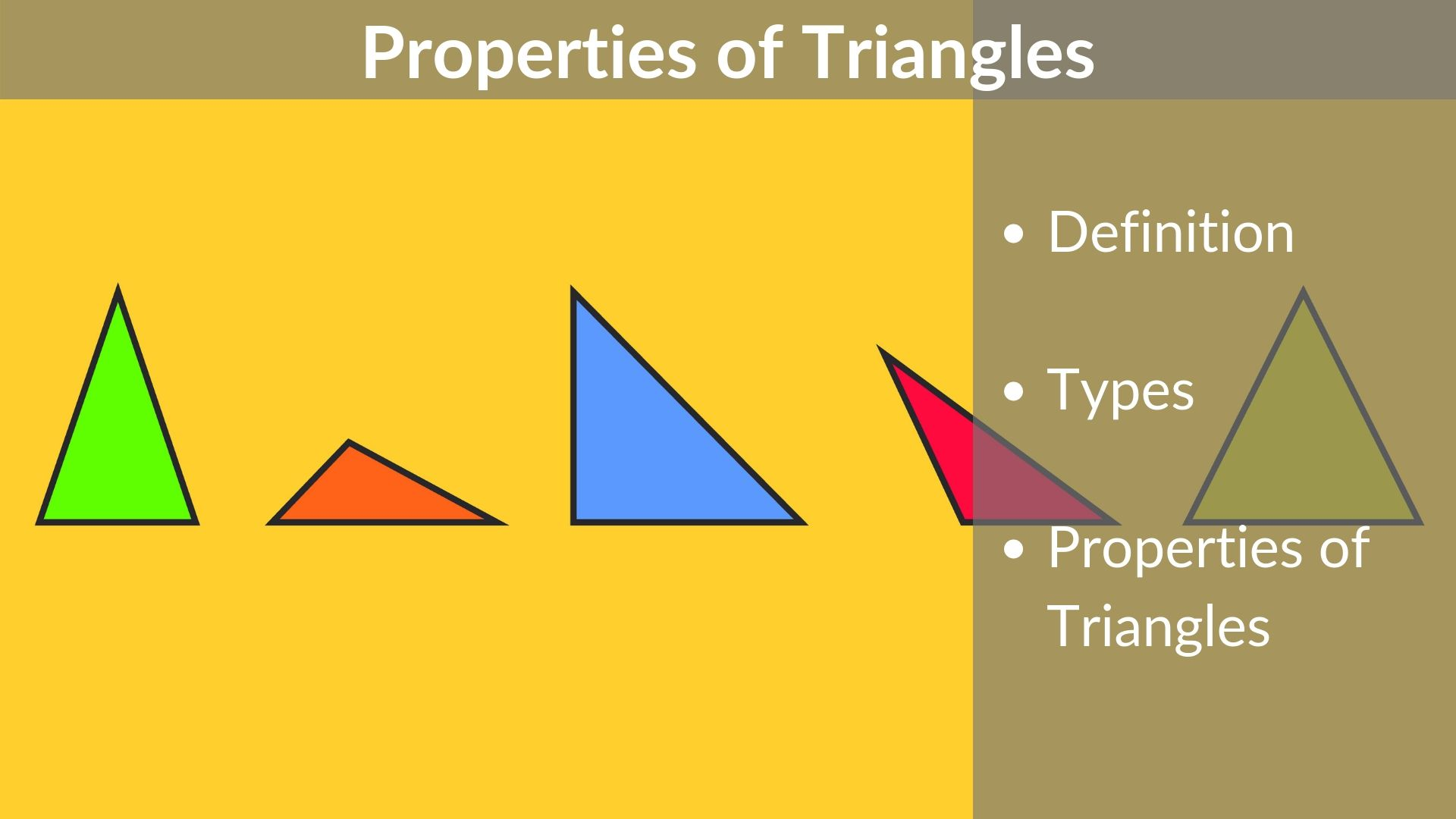# How to Prepare for GMAT Quant

A 10 minute read

1. Introduction If you are someone who has always been weak at math, then we understand how you’d be feeling about GMAT Quant questions and its syllabus. You were probably happy (and relieved!) to finally be done with all things mathematics when school ended. But now, the demon is back on your back!   But

Read More# Tips to score a Q50+ in the GMAT Quant Section

A 16 minute read

Among the many elements of your MBA application, the GMAT is the one that you have a great chance of acing, even if you have had no business with it so far. This is obviously not true for most other elements, such as having good work experience or having good undergrad grades. Those elements are

Read More# How to get a Q51 on the GMAT

A 16 minute read

Among the many elements of your MBA application, the GMAT is the one that you have a great chance of acing, even if you have had no business with it so far. This is obviously not true for most other elements, such as having good work experience or having good undergrad grades. Those elements are

Read More# Properties of Quadrilaterals – rectangle, square, parallelogram, rhombus, trapezium

A 7 minute read

In Euclidean geometry, a quadrilateral is a four-sided 2D figure whose sum of internal angles is 360°. The word quadrilateral is derived from two Latin words ‘quadri’ and ‘latus’ meaning four and side respectively. Therefore, identifying the properties of quadrilaterals is important when trying to distinguish them from other polygons. So, what are the properties

Read More# Properties of Triangle – types & formulas [Video & Practice]

A 7 minute read

In this article, we are going to learn about the simplest form of a polygon, a triangle. All polygons can be divided into triangles, or in other words, they are formed by combining two or more triangles. Thus, understanding the basic properties of a triangle and its types is essential. There are six types of

Read More# Lines and Angles – Definitions, Properties, Types, Practice Questions

A 7 minute read

Ancient mathematicians introduced the concept of lines to represent straight objects which had negligible width and depth. Considered as a breadth less length by Euclid, lines form the basis of Euclidean geometry. When two rays (part of a straight line) intersect each other in the same plane, they form an angle. The point of intersection

Read More# The figure above shows a drop-leaf table. With all four leaves down the tabletop is a square, and with all four leaves up the tabletop is a circle. What is the radius, in meters, of the tabletop when all four leaves are up? – OGQR 2020 Question #99 with Solution

A 2 minute read

OGQR 2020: Question No. 99 The figure above shows a drop-leaf table. With all four leaves down the tabletop is a square, and with all four leaves up the tabletop is a circle. What is the radius, in meters, of the tabletop when all four leaves are up? Source OGQR 2020 Type Problem Solving Topic

Read More# P is a particle on the circle shown above. What is the length of the path travelled by P in one complete revolution around the circle? – OGQR 2020 Question #212 with Solution

A 2 minute read

OGQR 2020: Question No. 212 P is a particle on the circle shown above. What is the length of the path traveled by P in one complete revolution around the circle? The diameter of the circle is 1.5 meters. The particle P moves in a clockwise direction at 0.5 meter per second. Source OGQR 2020 Type Data Sufficiency Topic Geometry/Word Problems Sub-Topic

Read More# If Car A took n hours to travel 2 miles and Car B took m hours to travel 3 miles, which of the following expresses the time it would take Car C, traveling at the average (arithmetic mean) of those rates, to travel 5 miles? – OGQR 2020 Question #79 with Solution

A < 1 minute read

OGQR 2020: Question No. 79 If Car A took n hours to travel 2 miles and Car B took m hours to travel 3 miles, which of the following expresses the time it would take Car C, traveling at the average (arithmetic mean) of those rates, to travel 5 miles? Source OGQR 2020 Type Problem

Read More# In a rectangular coordinate system, straight-line k passes through points (0, 0) and (3, 2). Which of the following are coordinates of a point on k? – OGQR 2020 Question #78 with Solution

A 2 minute read

OGQR 2020: Question No. 78 In a rectangular coordinate system, straight-line k passes through points (0, 0) and (3, 2). Which of the following are coordinates of a point on k? Source OGQR 2020 Type Problem Solving Topic Geometry Sub-Topic Coordinate Geometry Difficulty Easy-Medium Solution Given In this question, we are given In a rectangular

Read More